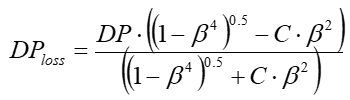Calculation of Pressure Loss - Intergraph Smart Instrumentation - 13.1 - Reference - Hexagon PPM

Integraph Smart Instrumentation Sizing Equations

Language
English (United States)
Product
Intergraph Smart Instrumentation
Search by Category
Reference
Smart Instrumentation Version
13.1
1. Calculate internal pipe diameter at flow temperature: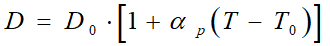2. Calculate correction factor for steam quality (for liguid, water, and steam, Fs=1):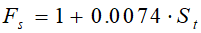3. Calculate orifice diameter at flow temperature.

1. For Venturi tubes and nozzles: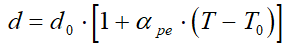2. For orifice plates: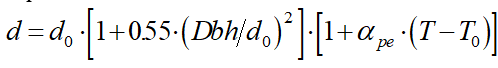4. Calculate pipe Reynolds number (W[kg/s]):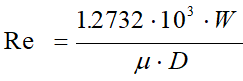5. Calculate b - ratio: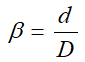6. Calculate discharge coefficient. See Appendix.

7. Calculate critical flow rate.

1. For liquid/water: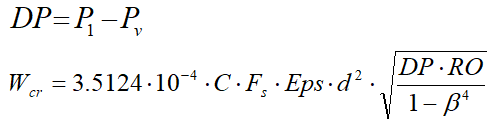2. For gas/steam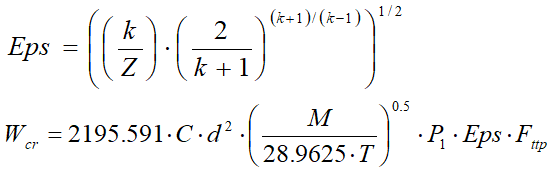8. Checking for critical flow and pressure loss calculation.

1. Case A - Critical flow: W>0.999*Wcr and W<1.001*Wcr.

For this case, pressure loss: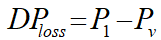2. Case B - Wrong data: W=>1.001*Wcr. Flow rate cannot be achieved, check data.

3. Case C- Sub-critical flow: W<=0.999*Wcr:

First estimate for differential range: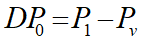4. Calculate discharge coefficient. See Appendix.

5. Calculate gas expansion factor (for liguid and water, Eps=1).

1. For Venturi tubes and nozzles: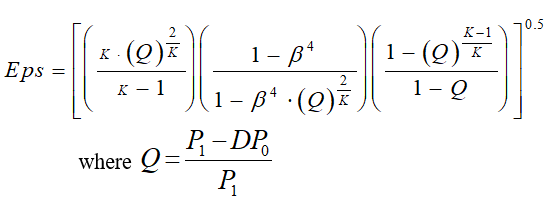2. For Orifice plates: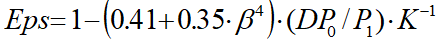6. Calculate new differential range (W[kg/s]):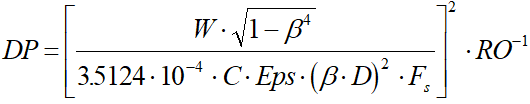7. Calculate the relative change in the differential range: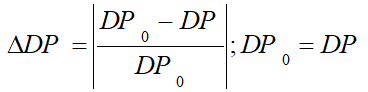8. Repeat steps e to g until the relative change in the DP is less than 0.0001.

9. Calculate pressure loss: